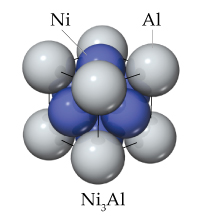# Problem: Ni3Al is used in the turbines of aircraft engines because of its strength and low density. Nickel metal has a cubic close-packed structure with a face-centered cubic unit cell, whereas Ni3Al has the ordered cubic structure shown in the figure below. The length of the cubic unit cell edge is 3.53 Ni3Al for nickel and 3.56 Ni3Al for Ni3Al. Use these data to calculate and compare the densities of these two materials.Give the calculated density of the Ni3Al alloy (ordered structure shown in the image with an edge length of 3.56 Å).

###### FREE Expert Solution
83% (336 ratings)
###### Problem Details

Ni3Al is used in the turbines of aircraft engines because of its strength and low density. Nickel metal has a cubic close-packed structure with a face-centered cubic unit cell, whereas Ni3Al has the ordered cubic structure shown in the figure below. The length of the cubic unit cell edge is 3.53 Ni3Al for nickel and 3.56 Ni3Al for Ni3Al. Use these data to calculate and compare the densities of these two materials.Give the calculated density of the Ni3Al alloy (ordered structure shown in the image with an edge length of 3.56 Å).